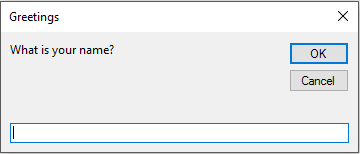# VBA InputBox Function

The VBA’s `InputBox` function is useful for obtaining a single piece of information from the user.

That information could be a value, a text string, or even a range address.

This is a good alternative to developing a `UserForm` when you need to get only one value.

## InputBox syntax

Here’s a simplified version of the syntax for the InputBox function:

``````' InputBox syntax
InputBox(prompt[, title][, default])
``````

The InputBox function accepts the arguments listed in below.

Arguments What it means
prompt The text displayed in the input box.
Title The text displayed in the input box’s title bar (optional).
Default The default value for the user’s input (optional)

## An InputBox example

Here’s an example showing how you can use the InputBox function:

``````' InputBox example
TheName = InputBox("What is your name?", "Greetings")
``````

When you execute this VBA statement, application displays the dialog box shown in below figure.

Notice that this example uses only the first two arguments and does not supply a default value.

When the user enters a value and clicks `OK`, the routine assigns the value to the variable `TheName`.Please note that VBA’s InputBox function always returns a `string`, so if you need to get a value, your code will need to do some additional checking.

The following example uses the InputBox function to get a number.

It uses the `IsNumeric` function to check whether the string is a number.

If the string does contain a number, all is fine.

If the user’s entry cannot be interpreted as a number, the code displays a message box.

``````' InputBox example
Sub GetDrawingSheetNumber()
Dim NumberOfSheets as String
Prompt = "How many sheets drawing have?"
NumberOfSheets = InputBox (Prompt)

If NumberOfSheets = "" Then Exit Sub
If (IsNumeric)NumberOfSheets Then
'......[Some code here]....
Else